Custom SearchGraphical Solution If there is a pair of numbers that can be substituted for x and y in two different equations, the pair form the coordinates of a point which lies on the graph of. each equation. The only way in which a point can lie on two lines simultaneously is for the point to be at the intersection of the lines. Therefore, the graphical solution of two simultaneous equations involves drawing their graphs and locating the point at which the graph lines intersect. For example, when we graph the equations x+y=33 and x-y = 5, as in figure 12-5, we see that they intersect in a single point. There is one pair of values comprising coordinates of that point (19, 14), and that pair of values satisfies both equations, as follows: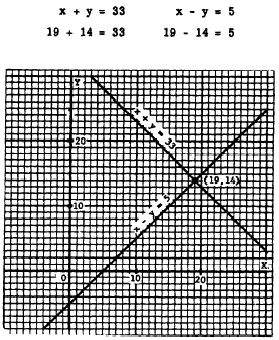Figure 12-5.-Graph of x + y = 33 and x - y = 5. This pair of numbers satisfies each equation. It is the only pair of numbers that satisfies the two equations simultaneously. The graphical method is a quick and simple means of finding an approximate solution of two simultaneous equations. Each equation is graphed, and the point of intersection of the two lines is read as accurately as possible. A high degree of accuracy can be obtained but this, of course, is dependent on the precision with which the lines are graphed and the amount of accuracy possible in reading the graph. Sometimes the graphical method is quite adequate for the purpose of the problem. Figure 12-6 shows the graphs of x + y = 11 and x-y= -3. The intersection appears to be the point (4, 7). Substituting x = 4 and y = 7 into the equations shows that this is the actual point of intersection, since this pair of numbers satisfies both equations.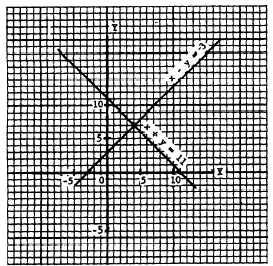Figure 12-6 - Graph of x + y = 11 and x - y = -3. The equations 7x - 8y = 2 and 4x + 3y = 5 are graphed in figure 12-7. The lines intersect where y is approximately 1/2 and x is approximately 5/6. Practice problems. Solve the following simultaneous systems graphically: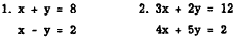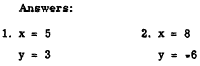Addition Method The addition method of solving systems of equations is illustrated in the following example: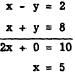The result in the foregoing example is obtained by adding the left member of the first equation to the left member of the second, and adding the right member of the first equation to the right member of the second. Having found the value of x, we substitute this value in either of the original equations to find the value of y, as follows: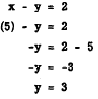Notice that the primary goal in the addition method is the elimination (temporarily) of ,one of the variables. If the coefficient of y is the same in both equations, except for its sign, adding the equations eliminates y as in the foregoing example. On the other hand, suppose that the coefficient of the variable which we desire to eliminate is exactly the same in both equations. In the following example, the coefficient of x is the same in both equations, including its sign: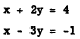Integrated Publishing, Inc. - A (SDVOSB) Service Disabled Veteran Owned Small Business